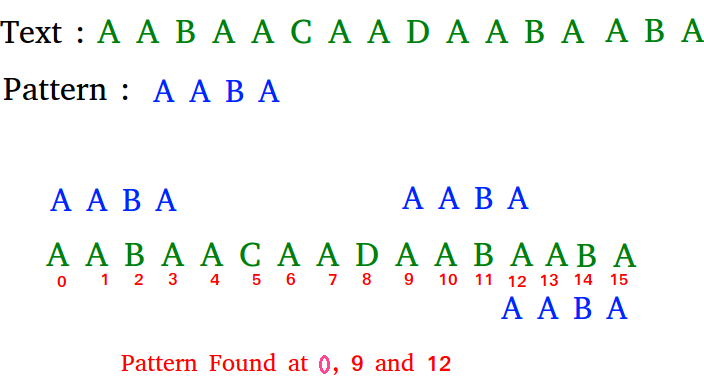Related Articles

# C# Program for KMP Algorithm for Pattern Searching

• Difficulty Level : Basic
• Last Updated : 11 Dec, 2018

Given a text txt[0..n-1] and a pattern pat[0..m-1], write a function search(char pat[], char txt[]) that prints all occurrences of pat[] in txt[]. You may assume that n > m.

Examples:

```Input:  txt[] = "THIS IS A TEST TEXT"
pat[] = "TEST"
Output: Pattern found at index 10

pat[] =  "AABA"
Output: Pattern found at index 0
Pattern found at index 9
Pattern found at index 12```

## Recommended: Please solve it on “PRACTICE ” first, before moving on to the solution.

Pattern searching is an important problem in computer science. When we do search for a string in notepad/word file or browser or database, pattern searching algorithms are used to show the search results.

## C#

 `// C# program for implementation of KMP pattern``// searching algorithm``using` `System;`` ` `class` `GFG {`` ` `    ``void` `KMPSearch(``string` `pat, ``string` `txt)``    ``{``        ``int` `M = pat.Length;``        ``int` `N = txt.Length;`` ` `        ``// create lps[] that will hold the longest``        ``// prefix suffix values for pattern``        ``int``[] lps = ``new` `int``[M];``        ``int` `j = 0; ``// index for pat[]`` ` `        ``// Preprocess the pattern (calculate lps[]``        ``// array)``        ``computeLPSArray(pat, M, lps);`` ` `        ``int` `i = 0; ``// index for txt[]``        ``while` `(i < N) {``            ``if` `(pat[j] == txt[i]) {``                ``j++;``                ``i++;``            ``}``            ``if` `(j == M) {``                ``Console.Write(``"Found pattern "``                              ``+ ``"at index "` `+ (i - j));``                ``j = lps[j - 1];``            ``}`` ` `            ``// mismatch after j matches``            ``else` `if` `(i < N && pat[j] != txt[i]) {``                ``// Do not match lps[0..lps[j-1]] characters,``                ``// they will match anyway``                ``if` `(j != 0)``                    ``j = lps[j - 1];``                ``else``                    ``i = i + 1;``            ``}``        ``}``    ``}`` ` `    ``void` `computeLPSArray(``string` `pat, ``int` `M, ``int``[] lps)``    ``{``        ``// length of the previous longest prefix suffix``        ``int` `len = 0;``        ``int` `i = 1;``        ``lps = 0; ``// lps is always 0`` ` `        ``// the loop calculates lps[i] for i = 1 to M-1``        ``while` `(i < M) {``            ``if` `(pat[i] == pat[len]) {``                ``len++;``                ``lps[i] = len;``                ``i++;``            ``}``            ``else` `// (pat[i] != pat[len])``            ``{``                ``// This is tricky. Consider the example.``                ``// AAACAAAA and i = 7. The idea is similar``                ``// to search step.``                ``if` `(len != 0) {``                    ``len = lps[len - 1];`` ` `                    ``// Also, note that we do not increment``                    ``// i here``                ``}``                ``else` `// if (len == 0)``                ``{``                    ``lps[i] = len;``                    ``i++;``                ``}``            ``}``        ``}``    ``}`` ` `    ``// Driver program to test above function``    ``public` `static` `void` `Main()``    ``{``        ``string` `txt = ``"ABABDABACDABABCABAB"``;``        ``string` `pat = ``"ABABCABAB"``;``        ``new` `GFG().KMPSearch(pat, txt);``    ``}``}`` ` `// This code has been contributed by Amit Khandelwal.`
Output:
```Found pattern at index 10
```

Please refer complete article on KMP Algorithm for Pattern Searching for more details!

My Personal Notes arrow_drop_up